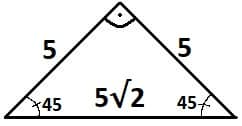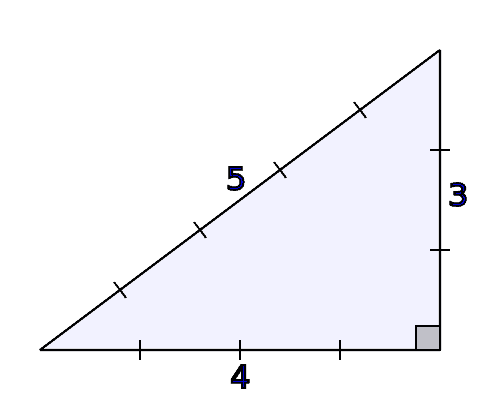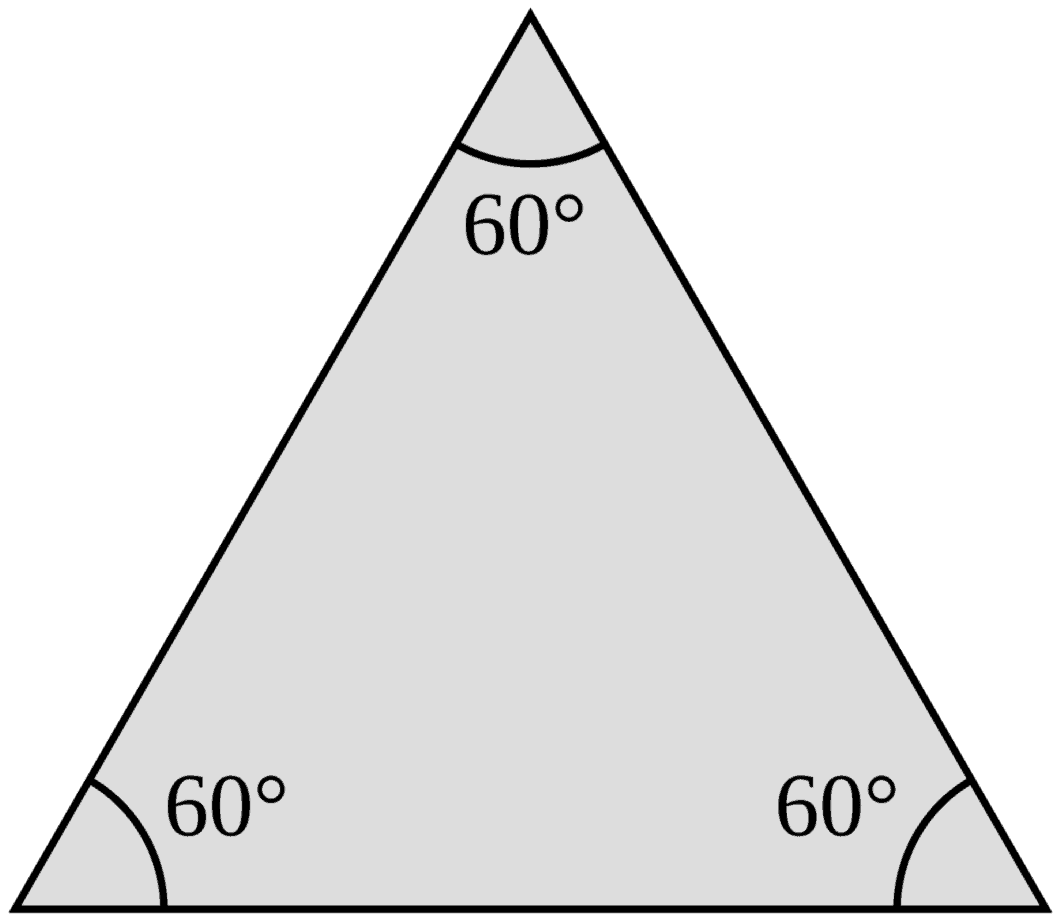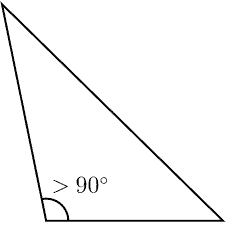# Right Triangles & Their Angles (7 Common Questions Answered)

Right triangles are important in many area of mathematics, including geometry, trigonometry, and calculus (that’s just for starters!)  However, there are lots of questions that you might have about right triangles.

So, what do you need to know about right triangles and their angles?  Some right triangles are isosceles or scalene, but no right triangle is equilateral, acute, or obtuse. Two right triangles can be similar, but not every pair of right triangles is similar. However, every pair of equilateral triangles or right isosceles triangles are similar.

Of course, it takes some work to think through questions about triangles and to prove these concepts.

In this article, we’ll take a closer look at some different types of right triangles.  We’ll also see which combinations are possible and which ones are not.

Let’s get started.

## All About Right Triangles & Their Angles (7 Common Questions Answered)

Right triangles come up often in geometry.  They are also central to trigonometry, which makes them useful in calculus as well.

However, there are lots of different types of triangles.  This raises certain questions about which combinations can occur.  Let’s answer some of those questions now.

### Can A Right Triangle Be Isosceles?

A right triangle can be isosceles.  However, not every right triangle is isosceles, and not every isosceles triangle is a right triangle.

Here are some important things to remember about a right isosceles triangle:

• Two sides are the same length – the two shortest sides (the legs) of the triangle have the same length, by definition of an isosceles triangle.
• The sides satisfy the equation for a Pythagorean Triple – since we have a right triangle, the side lengths a, b, and c of a right scalene triangle satisfy the equation a2 + b2 = c2, which comes from the Pythagorean Theorem.
• Two angles have the same measure of 45 degrees – the two smallest angles (across from the legs) of the triangle have the same measure, since their opposite sides have the same length.  As it turns out, their measure is 45 degrees (there is a proof of this below).
• One angle is 90 degrees – the largest angle (the right angle) is across from the largest side (the hypotenuse), and it measures 90 degrees, by definition of a right triangle.

Now let’s prove that the two smaller angles in a right isosceles triangle are 45 degrees.

First, remember that the three angles in any triangle add up to 180 degrees.  If the angles are x, y, and z, then:

• x + y + z = 180

Next, remember that the largest angle is 90 degrees (let’s say that angle is z).  Then:

• x + y + 90 = 180
• x + y = 90

Then, recall that the two smaller angles have the same measure.  This means that x = y, so:

• x + x = 90
• 2x = 90
• x = 45

Thus, the two smaller angles in a right isosceles triangle have a measure of 45 degrees.

This tells us that a right isosceles triangle has angles 45-45-90.  This gives us a specific ratio of side lengths: 1:1:√2.

So, if the shortest side of an isosceles triangle has a length of 10 feet, then the longest side (the hypotenuse) has a length of 10√2 feet.

Note: this means that all right isosceles triangles are similar.A right isosceles triangle has angles 45, 45, and 90 degrees. The sides also have lengths in the ratio 1:1:√2 .

Let’s look at an example of a right triangle that is isosceles – and then, a right triangle that is not isosceles.

#### Example 1: A Right Triangle That Is Isosceles

Consider a triangle with two sides that both have a length of 5 feet, which meet at a 90 degree angle.

Since the triangle has a 90 degree angle, we have a right triangle.  Since the two shorter sides of the right triangle are the same length, we have a right isosceles triangle.

Since the ratio of sides is 1:1:√2 and the length of the legs (the shorter sides) is 5, then the length of the longer side (the hypotenuse, across from the 90 degree angle) is 5√2.This right isosceles triangle has angles 45, 45, and 90 degrees. The sides also have lengths in the ratio 1:1:√2, after reducing from 5:5:5√2.

#### Example 2: A Right Triangle That Is Not Isosceles

Consider a triangle with two sides that have lengths of 3 feet and 4 feet, which meet at a 90 degree angle.  Since the triangle has a 90 degree angle, we know it is a right triangle.

However, since the two sides adjacent to the 90 degree angle (the legs) are not the same length, we know that this right triangle is not isosceles.

By the way, the 3rd and longest side of the triangle (the hypotenuse) has a length of 5 feet, by the Pythagorean Theorem with a = 3 and b = 4:

• a2 + b2 = c2
• 32 + 42 = c2
• 9 + 16 = c2
• 25 = c2
• 5 = cThis right triangle has side lengths 3, 4, and 5 (a Pythagorean Triple satisfying the equation a2 + b2 = c2). Thus, it is not isosceles, since all 3 sides have different lengths.

### Can A Right Triangle Be Scalene?

A right triangle can be scalene.  However, not every right triangle is scalene, and not every scalene triangle is a right triangle.

Here are some important things to remember about a right scalene triangle:

• One angle is 90 degrees – the largest angle (the right angle) is across from the largest side (the hypotenuse), and it measures 90 degrees, by definition of a right triangle.
• No two sides are the same length – by definition, the 3 sides of a scalene triangle have different lengths, so no two sides have the same length.  That is, if the side lengths are a, b, and c, then a ≠ b, a ≠ c, and b ≠ c.
• The sides satisfy the equation for a Pythagorean Triple – since we have a right triangle, the side lengths a, b, and c of a right scalene triangle satisfy the equation a2 + b2 = c2, which comes from the Pythagorean Theorem.

Let’s look at an example of a right triangle that is scalene – and then, a right triangle that is not scalene.

#### Example 1: A Right Triangle That Is Scalene

Consider the same triangle from our earlier example – the one with two sides that have lengths of 3 feet and 4 feet, which meet at a 90 degree angle.  Since the triangle has a 90 degree angle, we know it is a right triangle.

Since we have a right triangle, we know that the side lengths a, b, and c of the triangle satisfy the equation:

• a2 + b2 = c2

Since we have a = 3 and b = 4, we can solve for c (the length of the hypotenuse):

• 32 + 42 = c2
• 9 + 16 = c2
• 25 = c2
• 5 = c

So, the side lengths of this right triangle are 3, 4, and 5.  Since none of the sides are the same length, then the triangle is scalene.This right triangle has side lengths 3, 4, and 5 (a Pythagorean Triple satisfying the equation a2 + b2 = c2). Thus, it is scalene, since all 3 sides have different lengths.

#### Example 2: A Right Triangle That Is Not Scalene

Consider a triangle with two sides that both have a length of 5 feet, which meet at a 90 degree angle.

Since the triangle has a 90 degree angle, we have a right triangle.  Since the two shorter sides of the right triangle are the same length, we have a right isosceles triangle.

Since two sides have the same length, the triangle is not scalene.  In general, an isosceles triangle cannot be scalene.

In addition, any equilateral triangle is also not scalene, since all of the sides would have the same length.This right isosceles triangle has angles 45, 45, and 90 degrees. It is not scalene, since two sides have the same length of 5.

### Can A Right Triangle Be Equilateral?

A right triangle cannot be equilateral.  Remember that by definition, any equilateral triangle has 3 sides of the same length.

This means that the three angles of the triangle have the same measure.  This is because each angle is across from a side with a common length.

If the angle measure of all three angles in an equilateral triangle is x, then we have:

• x + x + x = 180  [the angles in a triangle add up to 180]
• 3x = 180
• x = 60

This implies that any equilateral triangle has three 60 degree angles.  This is true regardless of the length of its sides (so, all equilateral triangles are similar to one another).

Thus, an equilateral triangle cannot have a right angle, and a right triangle cannot be equilateral.Any equilateral triangle has three 60 degree angles (regardless of the length that the 3 sides share). An equilateral triangle cannot have a 90 degree angle, and no right triangle can be equilateral.

### Can A Right Triangle Be Acute?

A right triangle cannot be acute.  By definition, an acute triangle has three angles that each measure less than 90 degrees (that is, an acute triangle has three acute angles).

Since a right triangle has an angle that measures exactly 90 degrees, it cannot be acute.

However, note that any equilateral triangle is acute (since its three angles all measure 60 degrees).

### Can A Right Triangle Be Obtuse?

A right triangle cannot be obtuse.  By definition, an obtuse triangle has one angle that is greater than 90 degrees (an obtuse angle) and two angles that each measure less than 90 degrees (two acute angles).

Since a right triangle has an angle that measures exactly 90 degrees, it cannot be obtuse.

Note that no equilateral triangle is obtuse (since its three angles all measure 60 degrees).An obtuse triangle has one angle that is obtuse (greater than 90 degrees) and two angles that are acute (less than 90 degrees).

### Can Right Triangles Be Similar?

Right triangles can be similar, but two given right triangles are not always similar.

You may need to look at sides, angles, or both to determine if two triangles are similar or not.

Let’s look at some examples of right triangles that are similar, and some that are not.  (Note: two right triangles will always share at least one angle in common: the 90 degree angle).

#### Example 1: Two Right Triangles That Are Similar (Based On Side Lengths)

Consider two right triangles:

• Triangle A, with side lengths 3, 4, and 5.
• Triangle B, with side lengths 6, 8, and 10.

Note that the side lengths of Triangle B are a multiple of the side lengths of Triangle A:

• 10 = 2*5
• 8 = 2*4
• 6 = 2*3

This means that Triangles A and B are similar, based on their side lengths.

#### Example 2: Two Right Triangles That Are Similar (Based On Angles)

Consider two right triangles:

• Triangle A, with angles 90 and 60.
• Triangle B, with angles 60 and 30.

Note that the third angle of Triangle A must be 30 degrees, since the three angles must add up to 180.

Similarly, the third angle of Triangle B must be 90 degrees.

So, both Triangles A and B have angles of 30, 60, and 90 degrees.

This means that Triangles A and B are similar, based on their angles.

#### Example 3: Two Right Triangles That Are Not Similar (Based On Angles)

Consider two right triangles:

• Triangle A, with sides 3, 4, and 5.
• Triangle B, which is isosceles.

Note that Triangle A is scalene, since all three of its sides have different lengths.  This means that the two angles across from its shorter sides (the legs) must have different measures (specifically, neither one is 45 degrees).

Also, note that Triangle B must have angle measures 45, 45, and 90 degrees (based on what we proved earlier about right isosceles triangles).

So, the smaller angles in Triangle A do not have the same measure as the smaller angles in Triangle B.  Thus, Triangles A and B are not similar.

### Can Two Right Triangles Make A Rectangle?

Two right triangles can make a rectangle when placed together, if the base and height match up exactly.

For example, if you have two right triangles, each with a base of b and a height of h, then you can put them together to form a rectangle with length B and width H, giving a total area of bh.

In this case, each right triangle is exactly one half of the area of the entire rectangle.

## Conclusion

Now you know a little more about right triangles.  You also know the answers to some common questions about different types of right triangles.

You can learn about the Triangle Inequality here.

You can learn all about similar triangles here.

You can learn about uses of triangles in real life here.